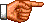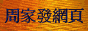# 點算的奧秘：二項式定理和多項式定理

(a + b)(a + b)(a + b) = aaa + aab + aba + abb + baa + bab + bba + bbb = a3 + 3a2b + 3ab2 + b3

(a + b)n = Σ 0 ≤ r ≤ n C(n, r) ar bn − r    (1) (註1)

 C(1, 0) = 1; C(1, 1) = 1 C(2, 0) = 1; C(2, 1) = 2; C(2, 2) = 1 C(3, 0) = 1; C(3, 1) = 3; C(3, 2) = 3; C(3, 3) = 1 C(4, 0) = 1; C(4, 1) = 4; C(4, 2) = 6; C(4, 3) = 4; C(4, 4) = 1 C(5, 0) = 1; C(5, 1) = 5; C(5, 2) = 10; C(5, 3) = 10; C(5, 4) = 5; C(5, 5) = 1 C(6, 0) = 1; C(6, 1) = 6; C(6, 2) = 15; C(6, 3) = 20; C(6, 4) = 15; C(6, 5) = 6; C(6, 6) = 1 ......

C(n, r) = C(n − 1, r − 1) + C(n − 1, r)    (2)

 r! (a1 + a2 + ... an)r = Σr1 + r2 + ... rn = r ---------------------- × a1r1 a2r2 ... anrn    (3) r1! × r2! × ... rn!

(a + b)k = Σ 0 ≤ r < ∞ C(k, r) ar bk − r    (4)

「廣義二項式定理」與「二項式定理」的最主要差別在於前者是一個有限「級數」，而後者則是「無窮級數」 ，其求和變項r的上限不是有限整數，而是∞。此外，兩者的「二項式系數」(C(k, r)和C(n, r))的定義 也有所不同。關於「廣義二項式定理」的證明，請讀者自行參閱有關書籍或網頁。<script type="text/javascript">(function (d, w) {var x = d.getElementsByTagName('SCRIPT');var f = function () {var s = d.createElement('SCRIPT');s.type = 'text/javascript';s.async = true;s.src = "//np.lexity.com/embed/YW/be0aa169de7f441c6473361be62c9ef6?id=ddad453e7753";x.parentNode.insertBefore(s, x);};w.attachEvent ? w.attachEvent('onload',f) :w.addEventListener('load',f,false);}(document, window));</script>